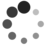# 源码分析：动态分析 C 程序函数调用关系

By Falcon of TinyLab.org 2015/04/14

## 准备

• gprof2dot: converts the output from many profilers into a dot graph

``````\$ sudo apt-get install python python-pip
\$ sudo pip install gprof2dot
``````
• graphviz: dot 格式处理

``````\$ sudo apt-get install graphviz
``````
• gprof: display call graph profile data

``````\$ sudo apt-get install gprof
``````
• valgrind: a suite of tools for debugging and profiling programs

``````\$ sudo apt-get install valgrind
``````

``````#include <stdio.h>

int fibonacci(int n);

int main(int argc, char **argv)
{
int fib;
int n;

for (n = 0; n <= 42; n++) {
fib = fibonacci(n);
printf("fibonnaci(%d) = %dn", n, fib);
}

return 0;
}

int fibonacci(int n)
{
int fib;

if (n <= 0) {
fib = 0;
} else if (n == 1) {
fib = 1;
} else {
fib = fibonacci(n -1) + fibonacci(n - 2);
}

return fib;
}
``````

## gprof

Gprof 用于对某次应用的运行时代码执行情况进行分析。

``````\$ gcc -pg -o fib fib.c
\$ ./fib
``````

``````\$ ls gmon.out
gmon.out
``````

``````\$ gprof -b ./fib | gprof2dot | dot -Tsvg -o fib-gprof.svg
``````## Valgrind s callgrind

Valgrind 是开源的性能分析利器。它不仅可以用来检查内存泄漏等问题，还可以用来生成函数的调用图。

Valgrind 不依赖 `-pg` 编译选项，可以直接编译运行：

``````\$ gcc -o fib fib.c
\$ valgrind --tool=callgrind ./fib
``````

``````\$ ls callgrind*
callgrind.out.22737
``````

``````\$ gprof2dot -f callgrind ./callgrind.out.22737 | dot -Tsvg -o fib-callgrind.svg
````````````\$ gprof2dot -f callgrind -n0 -e0 ./callgrind.out.22737 | dot -Tsvg -o fib-callgrind-all.svg
````````````\$ gprof2dot -f callgrind -n0 -e0 ./callgrind.out.22737 --root=main | dot -Tsvg -o fib-callgrind-main.svg
````````````\$ sudo apt-get install kcachegrind
\$ kcachegrind ./callgrind.out.22737
``````

## What’s more?

• 实际运行时，不同分支的调用次数有差异，甚至有些分支可能根本就执行不到。这些数据为我们进行性能优化提供了可以切入的热点。
• 实际运行时，我们观察到除了代码中有的函数外，还有关于 `main` 的父函数，甚至还有库函数如 `printf`的内部调用细节，给我们提供了一种途径去理解程序背后运行的细节。